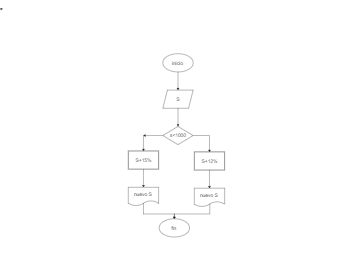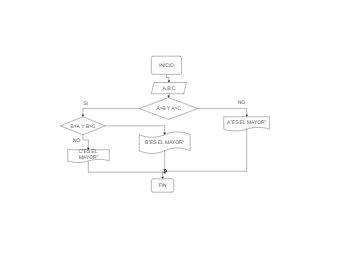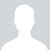## Booths Algorithm Flowchart

4
0
0
publish time: 2021-07-16Charlotte

As the below image illustrates, Booth's multiplication algorithm is a multiplication algorithm that multiplies two signed binary numbers in two's complement notation. It should be noted here that Booth Algorithm gives a procedure for multiplying binary integers in signed 2's complement representation inefficient way, i.e., less number of addition/subtractions required. The multiplicand is subtracted from the partial product upon encountering the first least significant 1 in a string of 1's in the multiplier. As the below algorithm suggests, the primary advantage of using the Booth Algorithm is that it handles both positive and negative multipliers uniformly. At the same time, it achieves efficiency in the number of additions required when the multiplier has a large block of 1's.

See More Related TemplatesHere is a math flowchart example. The flowchart is often used in representing algorithm, from which you could understand relative knowledge visually.
Math Flowchart Example
8
1
0Jose Luis Martinez Gonzaleztrabajo de diagrama de flujos
Dibujo1
17
0
0Sebastian Baron Lopez